# 2 什么是哈希？

Java 中所有的对象都有 Hash 方法

Java中的所有对象都继承 Object 类中定义的 hashCode() 函数的默认实现。 此函数通常通过将对象的内部地址转换为整数来生成哈希码，从而为所有不同的对象生成不同的哈希码。

# 3 HashMap 中的 Node 类

Map的定义是： 将键映射到值的对象。

    static class Node<K,V> implements Map.Entry<K,V> {
final int hash;// 记录hash值， 以便重hash时不需要再重新计算
final K key;
V value;
Node<K,V> next;
...// 其余的代码
}

# 4 键值对在 HashMap 中是如何存储的

transient Node<K,V>[] table;

static final int tableSizeFor(int cap) {
int n = cap - 1;// 如果不做该操作， 则如传入的 cap 是 2 的整数幂， 则返回值是预想的 2 倍
n |= n >>> 1;
n |= n >>> 2;
n |= n >>> 4;
n |= n >>> 8;
n |= n >>> 16;
return (n < 0) ? 1 : (n >= MAXIMUM_CAPACITY) ? MAXIMUM_CAPACITY : n + 1;
}

(n - 1) & hash

    01010011 00100101 01010100 00100101
&   00000000 00000000 00000000 00001111
----------------------------------
00000000 00000000 00000000 00000101    //高位全部归零，只保留末四位
// 保证了计算出的值小于数组的长度 n

    static final int hash(Object key) {
int h;
return (key == null) ? 0 : (h = key.hashCode()) ^ (h >>> 16);
}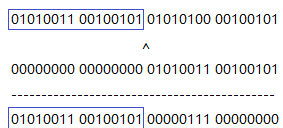# 5 哈希碰撞及其处理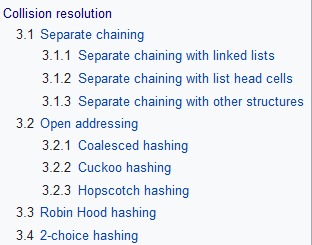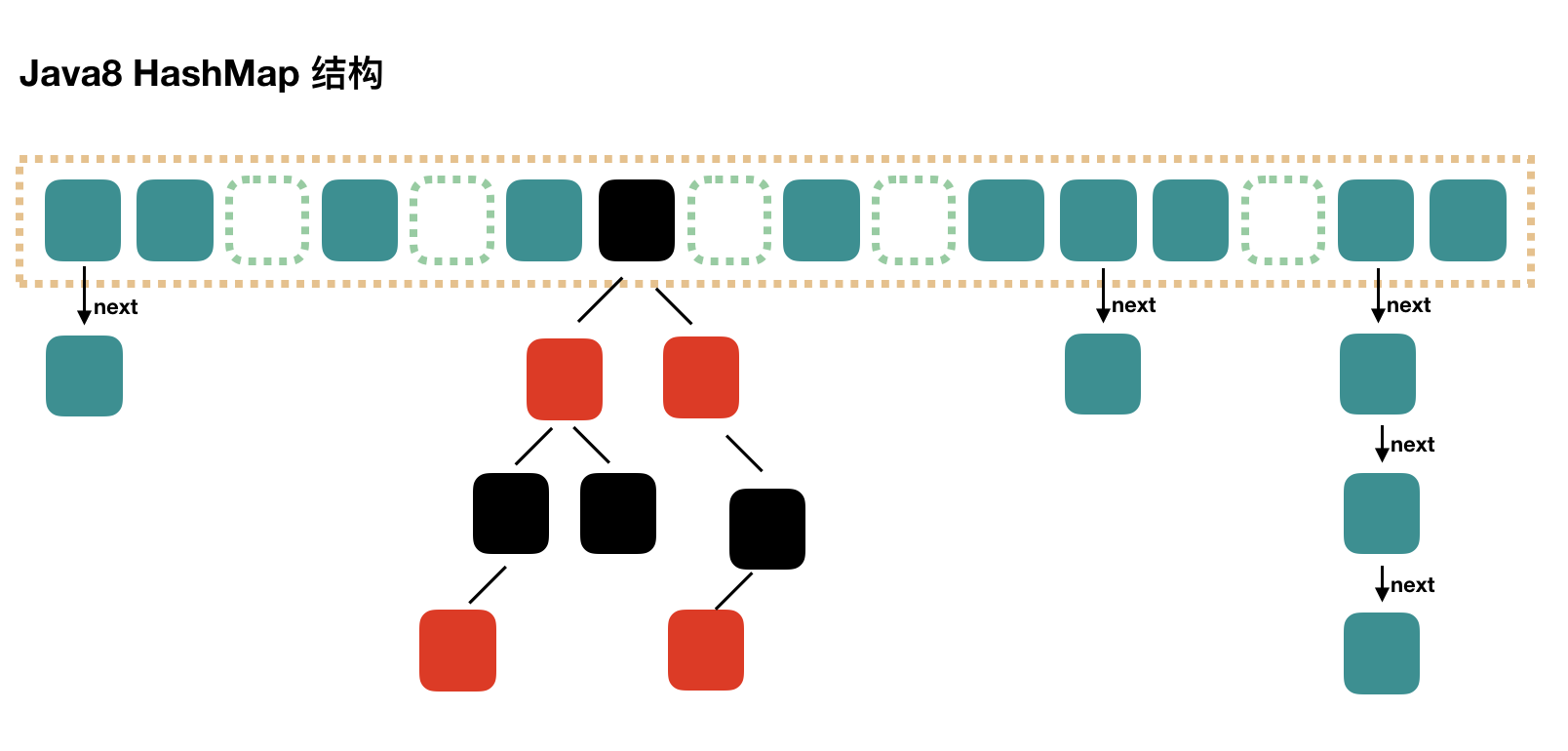static final int TREEIFY_THRESHOLD = 8;

static final int UNTREEIFY_THRESHOLD = 6;

# 6 HashMap 的初始化

 public HashMap();
public HashMap(int initialCapacity);
public HashMap(Map<? extends K, ? extends V> m);
public HashMap(int initialCapacity, float loadFactor); 

HashMap 中有四个构造函数， 大多是初始化容量和负载因子的操作。以 public HashMap(int initialCapacity, float loadFactor) 为例

public HashMap(int initialCapacity, float loadFactor) {
// 初始化的容量不能小于0
if (initialCapacity < 0)
throw new IllegalArgumentException("Illegal initial capacity: " +
initialCapacity);
// 初始化容量不大于最大容量
if (initialCapacity > MAXIMUM_CAPACITY)
initialCapacity = MAXIMUM_CAPACITY;
// 负载因子不能小于 0
throw new IllegalArgumentException("Illegal load factor: " +
this.threshold = tableSizeFor(initialCapacity);
}

# 7 HashMap 中哈希表的初始化或动态扩容

transient Node<K,V>[] table;

## 7.1 扩容发生的条件

threshold = loadFactor * capacity

threshold = loadFactor * capacity = 0.75 * 16 = 12

## 7.2 再谈容量为2的整数次幂和数组索引计算

index = (table.length - 1) & hash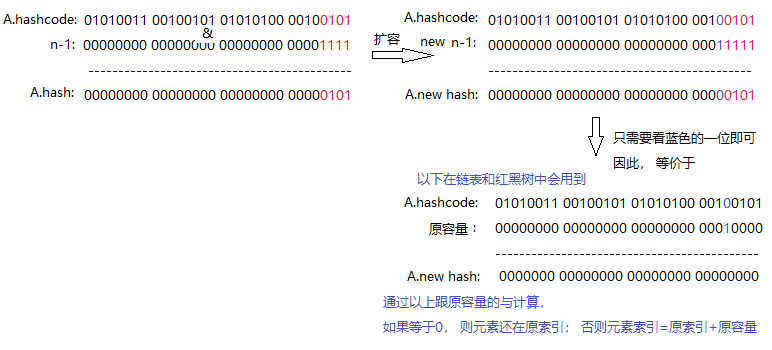## 7.3 步骤

1. 先判断是初始化还是扩容， 两者在计算 newCap和newThr 时会不一样
2. 计算扩容后的容量，临界值。
3. 将hashMap的临界值修改为扩容后的临界值
4. 根据扩容后的容量新建数组，然后将hashMap的table的引用指向新数组。
5. 将旧数组的元素复制到table中。在该过程中， 涉及到几种情况， 需要分开进行处理（只存有一个元素， 一般链表， 红黑树）

final Node<K, V>[] resize() {
//新建oldTab数组保存扩容前的数组table
Node<K, V>[] oldTab = table;
//获取原来数组的长度
int oldCap = (oldTab == null) ? 0 : oldTab.length;
//原来数组扩容的临界值
int oldThr = threshold;
int newCap, newThr = 0;
//如果扩容前的容量 > 0
if (oldCap > 0) {
//如果原来的数组长度大于最大值(2^30)
if (oldCap >= MAXIMUM_CAPACITY) {
//扩容临界值提高到正无穷
threshold = Integer.MAX_VALUE;
//无法进行扩容，返回原来的数组
return oldTab;
//如果现在容量的两倍小于MAXIMUM_CAPACITY且现在的容量大于DEFAULT_INITIAL_CAPACITY
} else if ((newCap = oldCap << 1) < MAXIMUM_CAPACITY &&
oldCap >= DEFAULT_INITIAL_CAPACITY)
//临界值变为原来的2倍
newThr = oldThr << 1;
} else if (oldThr > 0) //如果旧容量 <= 0，而且旧临界值 > 0
//数组的新容量设置为老数组扩容的临界值
newCap = oldThr;
else { //如果旧容量 <= 0，且旧临界值 <= 0，新容量扩充为默认初始化容量，新临界值为DEFAULT_LOAD_FACTOR * DEFAULT_INITIAL_CAPACITY
newCap = DEFAULT_INITIAL_CAPACITY;//新数组初始容量设置为默认值
newThr = (int) (DEFAULT_LOAD_FACTOR * DEFAULT_INITIAL_CAPACITY);//计算默认容量下的阈值
}
// 计算新的resize上限
if (newThr == 0) {//在当上面的条件判断中，只有是初始化时(oldCap=0, oldThr > 0)时，newThr == 0
//ft为临时临界值，下面会确定这个临界值是否合法，如果合法，那就是真正的临界值
float ft = (float) newCap * loadFactor;
//当新容量< MAXIMUM_CAPACITY且ft < (float)MAXIMUM_CAPACITY，新的临界值为ft，否则为Integer.MAX_VALUE
newThr = (newCap < MAXIMUM_CAPACITY && ft < (float) MAXIMUM_CAPACITY ?
(int) ft : Integer.MAX_VALUE);
}
//将扩容后hashMap的临界值设置为newThr
threshold = newThr;
//创建新的table，初始化容量为newCap
@SuppressWarnings({"rawtypes", "unchecked"})
Node<K, V>[] newTab = (Node<K, V>[]) new Node[newCap];
//修改hashMap的table为新建的newTab
table = newTab;
//如果旧table不为空，将旧table中的元素复制到新的table中
if (oldTab != null) {
//遍历旧哈希表的每个桶，将旧哈希表中的桶复制到新的哈希表中
for (int j = 0; j < oldCap; ++j) {
Node<K, V> e;
//如果旧桶不为null，使用e记录旧桶
if ((e = oldTab[j]) != null) {
//将旧桶置为null
oldTab[j] = null;
//如果旧桶中只有一个node
if (e.next == null)
//将e也就是oldTab[j]放入newTab中e.hash & (newCap - 1)的位置
newTab[e.hash & (newCap - 1)] = e;
//如果旧桶中的结构为红黑树
else if (e instanceof TreeNode)
//将树中的node分离
((TreeNode<K, V>) e).split(this, newTab, j, oldCap);
else {  //如果旧桶中的结构为链表,链表重排，jdk1.8做的一系列优化
Node<K, V> loHead = null, loTail = null;
Node<K, V> hiHead = null, hiTail = null;
Node<K, V> next;
//遍历整个链表中的节点
do {
next = e.next;
// 原索引
if ((e.hash & oldCap) == 0) {
if (loTail == null)
else
loTail.next = e;
loTail = e;
} else {// 原索引+oldCap
if (hiTail == null)
else
hiTail.next = e;
hiTail = e;
}
} while ((e = next) != null);
// 原索引放到bucket里
if (loTail != null) {
loTail.next = null;
}
// 原索引+oldCap放到bucket里
if (hiTail != null) {
hiTail.next = null;
newTab[j + oldCap] = hiHead;
}
}
}
}
}
return newTab;
}

# 8 HashMap.put() 函数内部是如何工作的？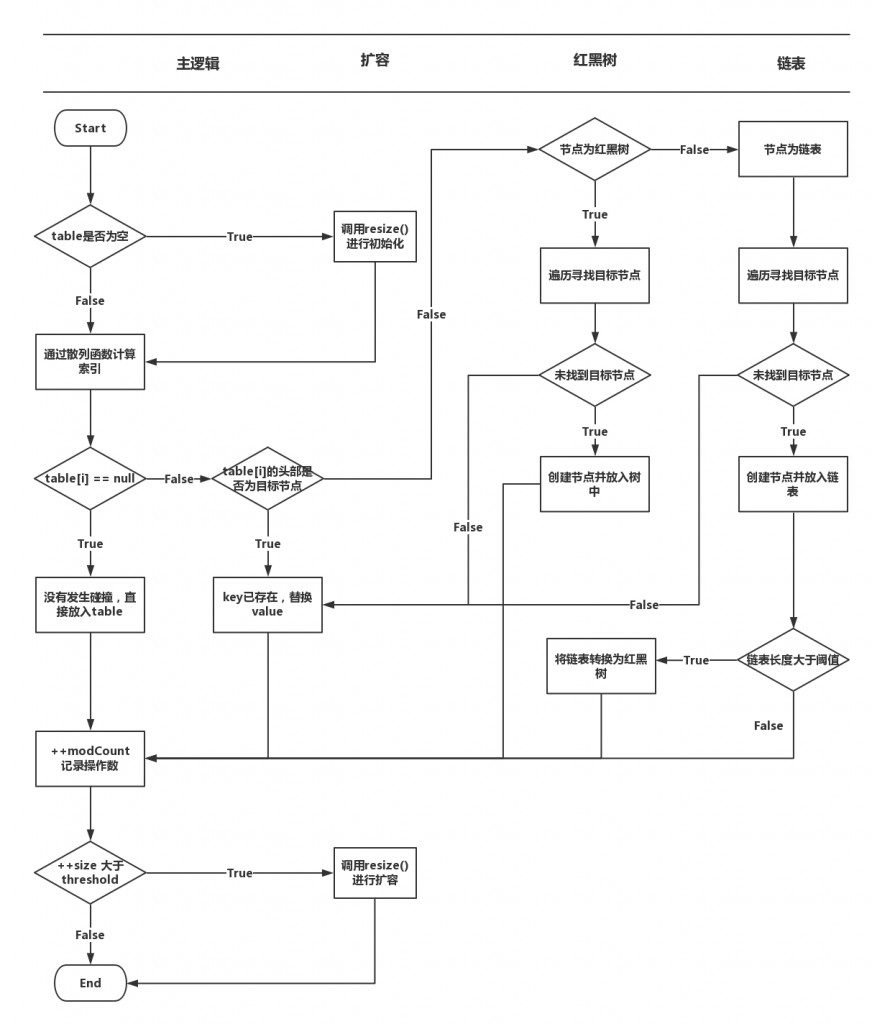/* @param hash         指定参数key的哈希值
* @param key          指定参数key
* @param value        指定参数value
* @param onlyIfAbsent 如果为true，即使指定参数key在map中已经存在，也不会替换value
* @param evict        如果为false，数组table在创建模式中
* @return 如果value被替换，则返回旧的value，否则返回null。当然，可能key对应的value就是null。
*/
final V putVal(int hash, K key, V value, boolean onlyIfAbsent,
boolean evict) {
Node<K, V>[] tab;
Node<K, V> p;
int n, i;
//如果哈希表为空，调用resize()创建一个哈希表，并用变量n记录哈希表长度
if ((tab = table) == null || (n = tab.length) == 0)
n = (tab = resize()).length;
/**
* 如果指定参数hash在表中没有对应的桶，即为没有碰撞
* Hash函数，(n - 1) & hash 计算key将被放置的槽位
* (n - 1) & hash 本质上是hash % n，位运算更快
*/
if ((p = tab[i = (n - 1) & hash]) == null)
//直接将键值对插入到map中即可
tab[i] = newNode(hash, key, value, null);
else {// 桶中已经存在元素
Node<K, V> e;
K k;
// 比较桶中第一个元素(数组中的结点)的hash值相等，key相等
if (p.hash == hash &&
((k = p.key) == key || (key != null && key.equals(k))))
// 将第一个元素赋值给e，用e来记录
e = p;
// 当前桶中无该键值对，且桶是红黑树结构，按照红黑树结构插入
else if (p instanceof TreeNode)
e = ((TreeNode<K, V>) p).putTreeVal(this, tab, hash, key, value);
// 当前桶中无该键值对，且桶是链表结构，按照链表结构插入到尾部
else {
for (int binCount = 0; ; ++binCount) {
// 遍历到链表尾部
if ((e = p.next) == null) {
p.next = newNode(hash, key, value, null);
// 检查链表长度是否达到阈值，达到将该槽位节点组织形式转为红黑树
if (binCount >= TREEIFY_THRESHOLD - 1) // -1 for 1st
treeifyBin(tab, hash);
break;
}
// 链表节点的<key, value>与put操作<key, value>相同时，不做重复操作，跳出循环
if (e.hash == hash &&
((k = e.key) == key || (key != null && key.equals(k))))
break;
p = e;
}
}
// 找到或新建一个key和hashCode与插入元素相等的键值对，进行put操作
if (e != null) { // existing mapping for key
// 记录e的value
V oldValue = e.value;
/**
* onlyIfAbsent为false或旧值为null时，允许替换旧值
* 否则无需替换
*/
if (!onlyIfAbsent || oldValue == null)
e.value = value;
// 访问后回调
afterNodeAccess(e);
// 返回旧值
return oldValue;
}
}
// 更新结构化修改信息
++modCount;
// 键值对数目超过阈值时，进行rehash
if (++size > threshold)
resize();
// 插入后回调
afterNodeInsertion(evict);
return null;
}


# 9 HashMap.get() 方法内部是如何工作的？

    /**
* 返回指定的key映射的value，如果value为null，则返回null
* get可以分为三个步骤：
* 1.通过hash(Object key)方法计算key的哈希值hash。
* 2.通过getNode( int hash, Object key)方法获取node。
* 3.如果node为null，返回null，否则返回node.value。
*
* @see #put(Object, Object)
*/
public V get(Object key) {
Node<K, V> e;
//根据key及其hash值查询node节点，如果存在，则返回该节点的value值
return (e = getNode(hash(key), key)) == null ? null : e.value;
}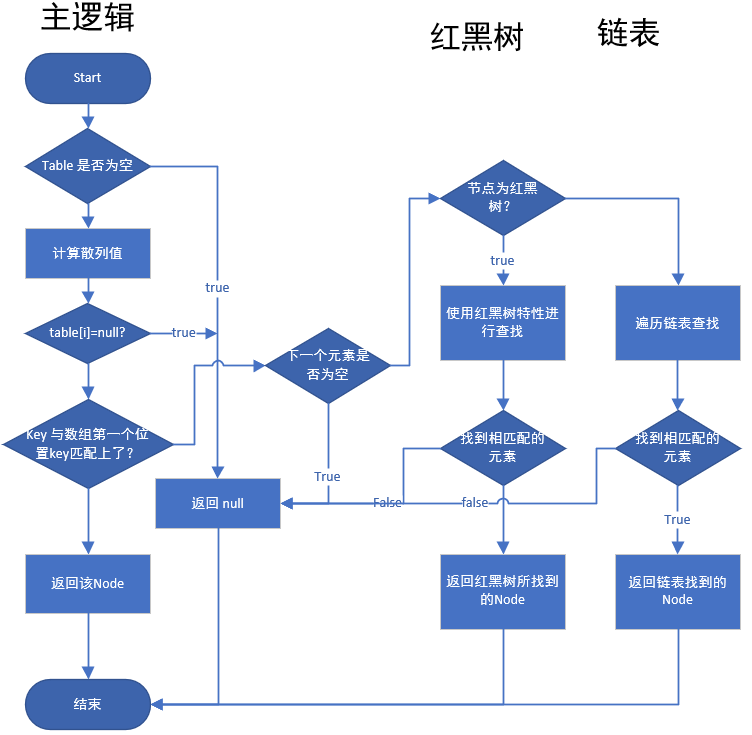/**
* @param hash 指定参数key的哈希值
* @param key  指定参数key
* @return 返回node，如果没有则返回null
*/
final Node<K, V> getNode(int hash, Object key) {
Node<K, V>[] tab;
Node<K, V> first, e;
int n;
K k;
//如果哈希表不为空，而且key对应的桶上不为空
if ((tab = table) != null && (n = tab.length) > 0 &&
(first = tab[(n - 1) & hash]) != null) {
//如果桶中的第一个节点就和指定参数hash和key匹配上了
if (first.hash == hash && // always check first node
((k = first.key) == key || (key != null && key.equals(k))))
//返回桶中的第一个节点
return first;
//如果桶中的第一个节点没有匹配上，而且有后续节点
if ((e = first.next) != null) {
//如果当前的桶采用红黑树，则调用红黑树的get方法去获取节点
if (first instanceof TreeNode)
return ((TreeNode<K, V>) first).getTreeNode(hash, key);
//如果当前的桶不采用红黑树，即桶中节点结构为链式结构
do {
//遍历链表，直到key匹配
if (e.hash == hash &&
((k = e.key) == key || (key != null && key.equals(k))))
return e;
} while ((e = e.next) != null);
}
}
//如果哈希表为空，或者没有找到节点，返回null
return null;
}